## ML Aggarwal Class 8 Solutions for ICSE Maths Chapter 1 Rational Numbers Ex 1.5

Question 1.
Represent the following rational numbers on the number line:
(i) $$\frac { 11 }{ 4 }$$
(ii) 4$$\frac { 3 }{ 5 }$$
(iii) $$\frac { -9 }{ 7 }$$
(iv) $$\frac { -2 }{ -5 }$$
Solution: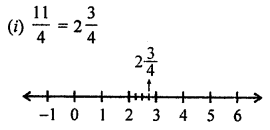Question 2.
Write the rational numbers for each point labelled with a letter: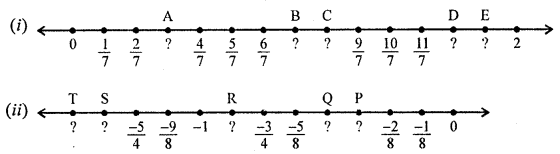Solution: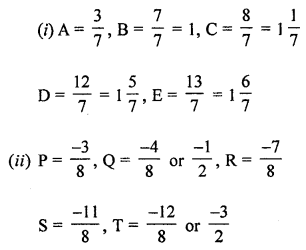Question 3.
Find twenty rational numbers between $$\frac { -3 }{ 7 }$$ and $$\frac { 2 }{ 3 }$$
Solution:
20 rational numbers between $$\frac { -3 }{ 7 }$$ and $$\frac { 2 }{ 3 }$$
LCM of 7, 3 = 21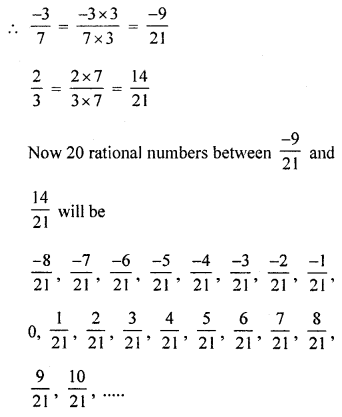Question 4.
Find six rational numbers between $$\frac { -1 }{ 2 }$$ and $$\frac { 5 }{ 4 }$$
Solution:
6 rational numbers between $$\frac { -1 }{ 2 }$$ and $$\frac { 5 }{ 4 }$$
LCM of 2, 4 = 4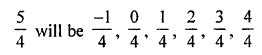Question 5.
Find three rational numbers between -2 and -1.
Solution:
3 rational numbers between -2 and -1
First rational number = $$\frac { 1 }{ 2 }$$ (-1 – 2) = $$\frac { -3 }{ 2 }$$
Second rational number between -2 and $$\frac { -3 }{ 2 }$$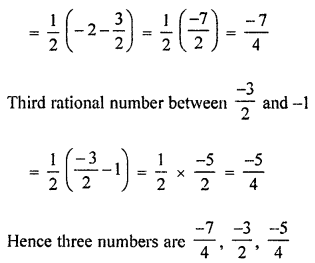Question 6.
Write ten rational numbers which are greater than 0.
Solution:
Ten rational numbers which are greater than 0.
There can be the infinite number of a rational number greater than 1.
We shall take only 10 to rational numbers such asQuestion 7.
Write five rational numbers which are smaller than -4.
Solution:
5 rational numbers which are smaller than -4.
These can be infinite numbers of rational numbers smaller than -4.
We shall take only 5 rational numbers such as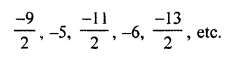Question 8.
Identify the rational number which is different from the other three. Explain your reasoning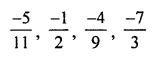Solution: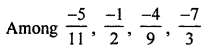$$\frac { -7 }{ 3 }$$ is different as its denominator is less than its numerator,
in others, denominators are greater than their numerators respectively.